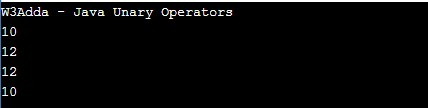# Java Unary Operators

In this tutorial you will learn about the Java Unary Operators and its application with practical example.

## Java Unary Operators (post and pre)

In Java, ++ and — are known as increment and decrement operators respectively. These are unary operators which means they work on a single operand. ++ adds 1 to operand and — subtracts 1 to operand respectively. When ++ is used as prefix(like: ++i), ++i will increment the value of i and then return it but, if ++ is used as postfix(like: i++), operator will return the value of operand first and then only increment it.

Operator Example Description
++ [prefix] ++a The value of an increment
++ [postfix] a++ The value of a before the increment
— [prefix] –a The value of an after decrement
— [postfix] a– The value of a before the decrement

Example:-

When you run the above java program, you will see the following output.

Output:-In this tutorial we have learn about the Java Unary Operators and its application with practical example. I hope you will like this tutorial.